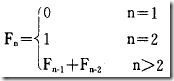# 斐波那契数列----C 语言学习1，使用递归方法：

int Fabonacci(int n)
{
if(n <= 2)
return n - 1;
else
return (Fabonacci(n - 1) + Fabonacci(n - 2));
}

2，使用 for 循环，输出了前 n 个斐波那契数列：

int _Fabonacci(int n)//输出前 n 个数
{
int i, a, b, c;
a = 0;
b = 1;
printf("%10d%10d", a, b);/*输出前两个数*/
for(i = 3; i <= n; i ++)
{
c = a + b;
printf("%10d", c);
if(i % 5 == 0)
printf("\n");/*每行输出 5 个*/
a = b;
b = c;/*项移动*/
}
printf("\n");
}
posted @ 2010-10-18 17:06  无墨来点睛  Views(2137)  Comments(0Edit  收藏  举报